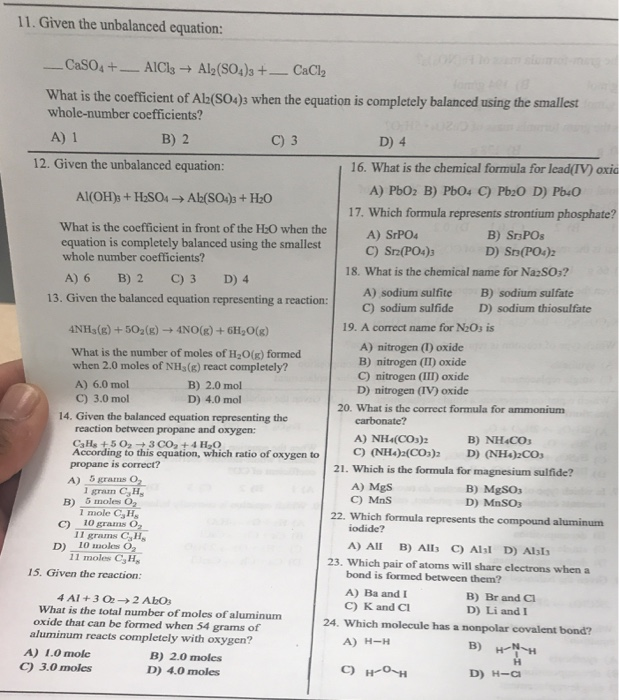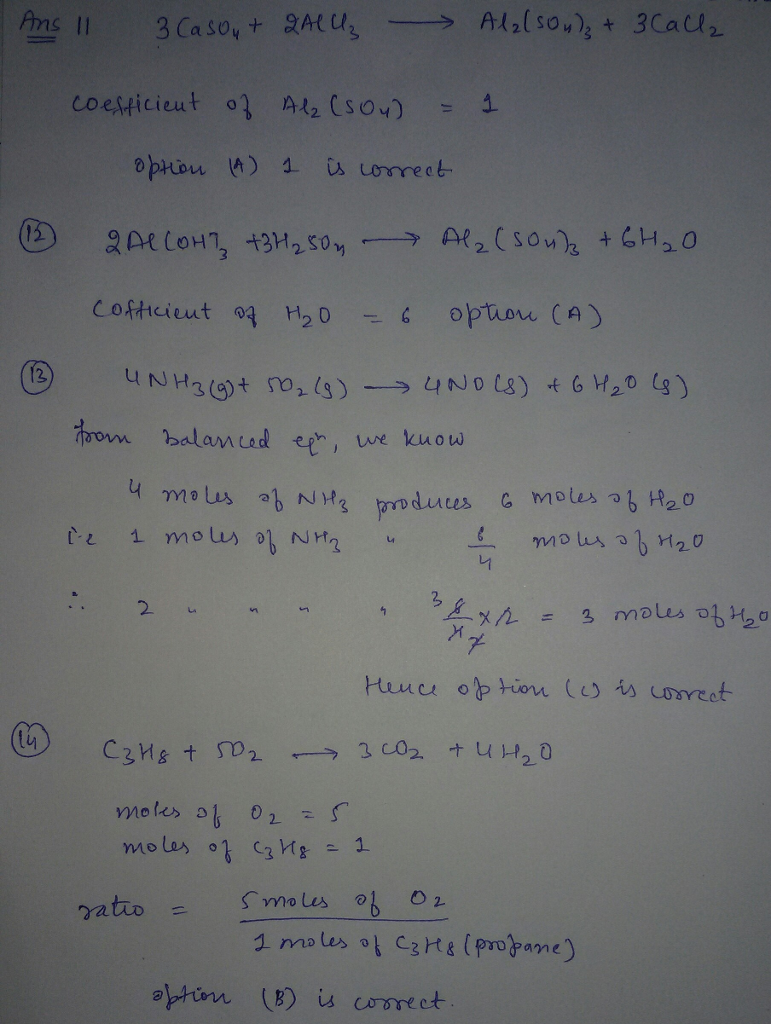# Question & Answer: Given the unbalanced equation: _____ CaSO_4 + _____ AlCl_3 rightarrow Al_2(SO_4)_3 +…..Given the unbalanced equation: _____ CaSO_4 + _____ AlCl_3 rightarrow Al_2(SO_4)_3 + _____ CaCl_2 What is the coefficient of Al_2(SO_4)_3 when the equation is completely balanced using the smallest whole-number coefficients? A) 1 B) 2 C) 3 D) 4 Given the unbalanced equation: Al(OH)_3 + H_2SO_4 rightarrow Al_2(SO_4)_3 + H_2O What is the coefficient in front of the H_2O when the equation is completely balanced using the smallest whole number coefficients? A) 6 B) 2 C) 3 D) 4 Given the balanced equation representing a reaction: 4NH_3(g) + 5O_2(g) rightarrow 4NO(g) + 6H_2O(g) What is the number of moles of H_2O(g) formed when 2.0 moles of NH_3(g) react completely? A) 6.0 mol B) 2.0 mol C) 3.0 mol D) 4.0 mol Given the balanced equation representing the reaction between propane and oxygen: C_3H_5 + 5 O_2 rightarrow 3 CO_2 + 4 H_2O According to this equation, which ratio of oxygen to propane is correct? A) 5 grams O_2/1 gram C_3H_8 B) 5 moles O_2/1 mole C_3H_8 C) 10 grams O_2/11 grams C_3H_8 D) 10 moles O_2/11 moles C_3H_8 Given the reaction: 4 Al + 3 O_2 rightarrow 2 Al_2O_3 What is the total number of moles of aluminum oxide that can be formed when 54 grams of aluminum reacts completely with oxygen? A) 1.0 moles B) 2.0 moles C) 3.0 moles D) 4.0 moles What is the chemical formula for lead (IV) oxide A) PbO_2 B) PbO_4 C) Pb_2O D) Pb_4O Which formula represents strontium phosphate? A) SrPO_4 B) Sr_3PO_8 C) Sr_2(PO_4)_3 D) Sr_3(PO_4)_2 What is the chemical name for Na_2SO_3? A) sodium sulfite B) sodium sulfate C) sodium sulfide D) sodium thiosulfate A correct name for N_2O_3 is A) nitrogen (I) oxide B) nitrogen (II) oxide C) nitrogen (III) oxide D) nitrogen (IV) oxide What is the correct formula for ammonium carbonate? A) NH_4(CO_3)_2 B) NH_4CO_3 C) (NH_4)_2(CO_3)_2 D) (NH_4)_2CO_3 Which is the formula for magnesium sulfate? A) MgS B) MgSO_3 C) MnS D) MnSO_3 Which formula represents the compound aluminum iodide? A) AII B) AII_3 C) AI_3I D) AI_3I_3 Which pair of atoms will share electrons when a bond is formed between them? A) Ba and I B) Br and Cl C) K and Cl D) Li and I Which molecule has a nonpolar covalent bond?